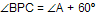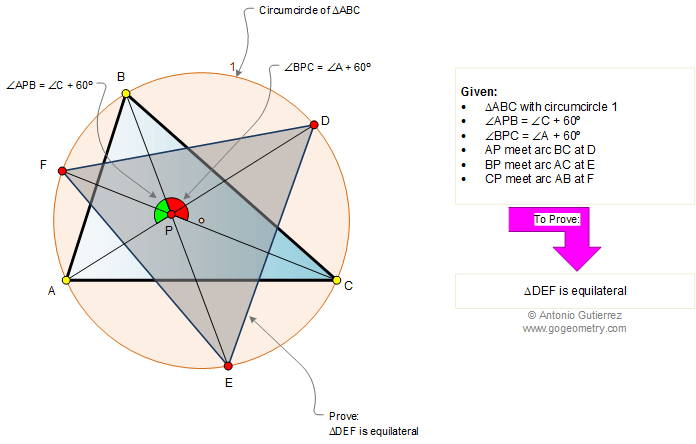Problem 366. Scalene triangle, Circumcircle, Angles, 60 Degrees, Equilateral triangle. Level: High School, College, SAT Prep. The figure shows a triangle ABC inscribed in a circle 1 (circumcircle) and an interior point P so thatand. Lines AP, BP, and CP intersect circle 1 at points D, E, and F, respectively. Prove that triangle DEF is equilateral.Recent Additions